## Factors Influencing the Sparkover Voltage of Sphere Gaps:

Various factors that affect the sparkover voltage of a sphere gap are:

• nearby earthed objects,
• atmospheric conditions and humidity,
• polarity and rise time of voltage waveforms.

### 1.Effect of nearby earthed objects:

The effect of nearby earthed objects was investigated by Kuffel by enclosing the earthed sphere inside an earthed cylinder. It was observed that the sparkover voltage is reduced. The reduction was observed to be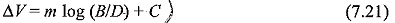where,

ΔV = percentage reduction,

B = diameter of earthed enclosing cylinder,

D = diameter of the spheres,

S = spacing, and m and C are constants.

The reduction was less than 2% for S/D ≤ 0.5 and B/D ≥ 0.8. Even for S/D ≈ 1.0 and B/D ≥ 1.0 the reduction was only 3%. Hence, if the specifications regarding the clearances are closely observed the error is within the tolerances and accuracy specified. The variation of breakdown voltage with A/D ratio is given in Figs 7.20a and b for a 50 cm sphere gap. The reduction in voltage is within the accuracy limits, if S/D is kept less than 0.6A in the above ratio A is the distance from sparking point to horizontal ground plane (also shown in Fig. 7.20).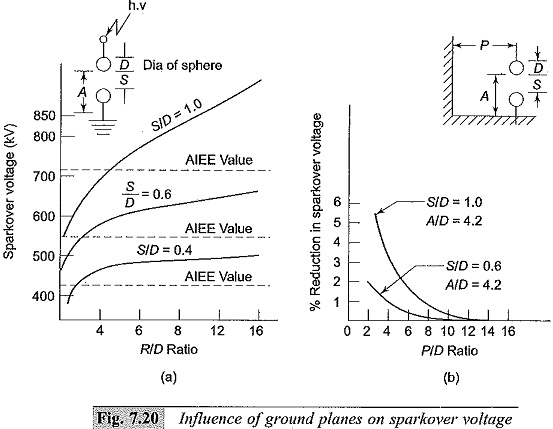### 2.Effect of atmospheric conditions:

The sparkover voltages of a spark gap depends on the air density which varies with the changes in both temperature and pressure. If the sparkover voltage is V under test conditions Temperature T and pressure p torr and if the sparkover voltage is V0 under standard conditions of temperature T= 20°C and pressure p = 760 torr, then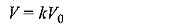where k is a function of the air density factor d, given by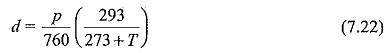The relationship between d and k is given in Table 7.6.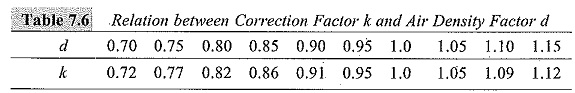The sparkover voltage increases with humidity. The increase is about 2 to 3% over normal humidity range of 8 g/m3 to 15 g/m3. The influence of humidity on sparkover voltage of a 25 cm sphere gap for 1 cm spacing is presented in Fig. 7.21.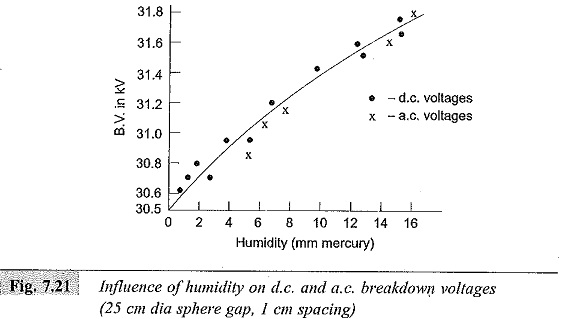Hence, it may be concluded that (i) the humidity effect increases with the size of spheres and is maximum for uniform field gaps, and (ii) the sparkover voltage increases with the partial pressure of water vapour in air, and for a given humidity condition, the change in sparkover voltage increases with the gap length. As the change in sparkover voltage with humidity is within 3%, no correction is normally given for humidity.